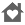# Finite Math ProEveryone
5
You're taking Finite Mathematics, and you don't have this app? Give yourself an edge!

"Finite Math Pro" contains a rich collection of tutorials, examples, and solvers for the following topics:

Points and Lines:

- Solvers and tutorials to find slopes, intercepts, equations of lines, and graphs of lines
- System solver for 2 variables and 2 equations
- Tutorials for solving systems by graphing, substitution, and linear combinations

Linear Regression:

- Solver to find the best fit line and correlation coefficient for up to 30 points
- Linear regression tutorial and example

Matrices:

- Adding, subtracting, and multiplying matrices
- Identity matrices
- Solvers and tutorials to find determinants/inverses of 2 x 2 and 3 x 3 matrices

Linear Programming (graphical method):

- Tutorial
- Example with step-by-step solution

Probability:

- Formulas
- Survey Results
- Venn Diagrams
- Bayes' Theorem
- Permutations
- Combinations
- Balls in Urns
- Independent Events
- Poker, Roulette, Dice, Lottery, General Card Problems

Normal Distributions:

- Solve for area under a bell curve without using Z-score tables
- Solve for value on bell curve given area without using Z-score tables
- Examples and tutorials

Compound Interest:

- Solve for amount, principle, time or rate
- Examples and tutorials

If you like my app, please leave a review! If you don't like my app, please email me with your constructive feedback!

I welcome all comments, questions, and suggestions:

lfeldman65@gmail.com

Please check out my other Android apps:

Algebra Pro
ACT Math Prep
Calculus Pro
Geometry Pro
Math Pro (6 math apps for \$1.99!)
PreCalculus Pro
Probability Pro
SAT Math Prep
Statistics Pro
Collapse

Review Policy
3.0
5 total
5
4
3
2
1

## What's New

Had to change the keyboard to alpha-numeric because some devices don't show a decimal point or negative sign otherwise. Believe me, I'm not happy about it either.
Collapse

Eligible for Family LibraryEligible if bought after 7/2/2016. Learn More
Updated
June 12, 2014
Size
672k
Installs
100+
Current Version
1.8
Requires Android
2.1 and up
Content Rating
Everyone
Permissions
Offered By
Larry Feldman
Developer
577 W. Bobier Drive, #202 Vista, CA 92083